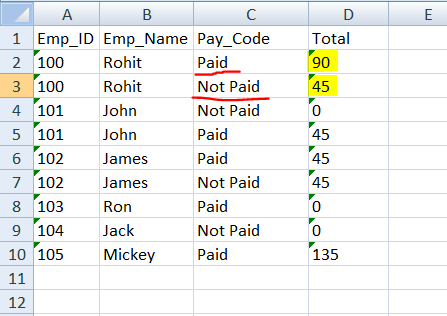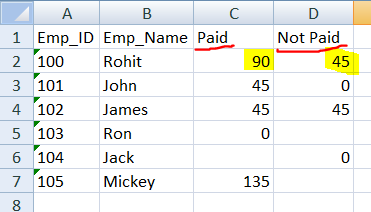# Create column as per Column's value and add data for same Employee in single row

Hi,

I want to read rows and create a new column as per Column C’s value (e.g. Paid, Not Paid) and add the value in a single row for the same Emp_ID. I’ve attached my Input sheet and I want the data like the Output screenshot.

Input:Output:@RohitK
Have a try on following

Prepare with build datatable the target data Column structure - dtResult
Cols: Emp_ID, EMP_Name, Paid, NotPaid
do not add any rows on it, we just do need the structure

Assign activity
Left side: dtResult

(From d in YourDataTableVar.AsEnumerable
Group d by k=d(“Emp_ID”).toString.Trim intor grp=Group
Let rp = grp.Where(Function (r1) r1(“Pay_Code”).toString.Trim.Equals(“Paid”))
Let rnp = grp.Where(Function (r2) r2(“Pay_Code”).toString.Trim.Equals(“Not Paid”))
Ler vp = rp.Select(Function (v1) v1(“Total”).toString).DefaultIfEmpty(“”).First()
Ler vnp = rnp.Select(Function (v2) v2(“Total”).toString).DefaultIfEmpty(“”).First()
Let ra = new Object(){k, grp.First()(1), vp,vnp}
Let us know if it does not work as expected or if the code needs more explanation@Hiba_B It worked as I wanted. Thank you so much.